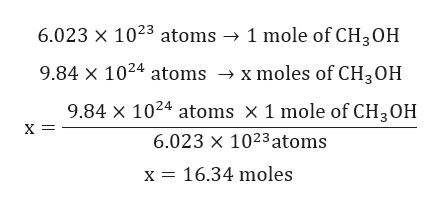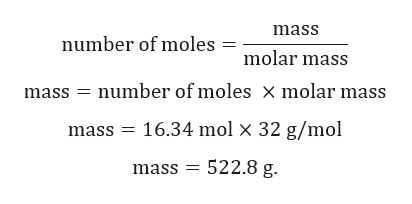What is the mass, in grams, of 9.84x1024 molecules of methanol (CH3OH)?

Question

What is the mass, in grams, of 9.84x1024 molecules of methanol (CH3OH)?

Step 1

Given:

Number of atoms = 9.84x1024 atoms.

Molar mass of CH3OH = 32 g/mol.

Step 2

Calculation for number of moles of CH3OH:

1 mole of any substance contain 6.023x1023 atoms.help_outlineImage Transcriptionclose6.023 x 1023 atoms -> 1 mole of CH3OH 9.84 x 1024 atoms -» x moles of CH3OH 9.84 X 1024 atoms X 1 mole of CH3OH х — 6.023 x 1023atoms x 16.34 moles fullscreen
Step 3

Calculation for mass of ...help_outlineImage Transcriptionclosemass number of moles molar mass mass number of moles X molar mass 16.34 mol x 32 g/mol mass mass 522.8 g. fullscreen

Want to see the full answer?

See Solution

Want to see this answer and more?

Our solutions are written by experts, many with advanced degrees, and available 24/7

See Solution
Tagged in

Chemistry